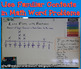# Fraction Line Plot WorksheetsSubject
Resource Type
File Type

PDF

(2 MB|4 pages)
Standards
Also included in:
1. Do you want a comprehensive set of Fraction Line Plot resources? This bundle contains task cards, worksheets (perfect for homework or seat work) and an open ended project for higher level thinking. With fun themes such as measuring the sugar in junk food, "how old were you when" line plots, and su
\$7.00
\$4.99
Save \$2.01
• Product Description
• Standards

Do your students need more practice learning to make a line plot to display a data set of measurements in fractions of a unit? I know when I went over the Common Core Standards CCSS Math 4.MD.B.4 had me scratching my head. We had NO resources to cover it. So I made my own!

These two worksheets use contexts that fourth graders are likely to be familiar with when it comes to measurement units and fractional parts. The types of questions vary between reading a line plot, creating a line plot with given data, subtracting fractions with like denominators, and justifying their answers. Answer keys are included.

Disclaimer Statement:

Any claims of correlation or alignment to the CCSS are solely those of Amber Thomas and have not been evaluated or endorsed by the NGA.

Amber Thomas is the sole creator of this product and does not claim endorsement or association with the creators of the CCSS.

✨✨✨ Are you looking for more ways to help your students deeply understand fractions? I have a whole range of fractions items here! Check out these popular products ✨✨✨
Chocolate Fractions: Equivalent Fractions Activity
Common Fractions and Improper Fractions Font
Equivalent Fractions Activities 3 in One Bundle
Fraction Line Plot Bundle
Fraction Line Plots: Peer Surveys Open Ended Project
Fraction Number Line Game: Long Jump
Fraction Number Line Worksheets
Fraction of a Set Task Cards Bundle of 3
Fractions Font and Fraction Circle Graphics {Value Pack}
Line Up! Compare and Order Fractions
Math Fractions Games for Kids: Bingo
Using One Half as a Benchmark Fraction: Coloring Page

Make a line plot to display a data set of measurements in fractions of a unit (1/2, 1/4, 1/8). Use operations on fractions for this grade to solve problems involving information presented in line plots. For example, given different measurements of liquid in identical beakers, find the amount of liquid each beaker would contain if the total amount in all the beakers were redistributed equally.
Make a line plot to display a data set of measurements in fractions of a unit (1/2, 1/4, 1/8). Solve problems involving addition and subtraction of fractions by using information presented in line plots. For example, from a line plot find and interpret the difference in length between the longest and shortest specimens in an insect collection.
Total Pages
4 pages
Included
Teaching Duration
45 minutes
Report this Resource to TpT
Reported resources will be reviewed by our team. Report this resource to let us know if this resource violates TpT’s content guidelines.